Tamil Nadu Board of Secondary EducationSSLC (English Medium) Class 6th

# Tamil Nadu Board Samacheer Kalvi solutions for Class 6th Mathematics Term 3 Answers Guide chapter 2 - Integers [Latest edition]

#### Chapters## Chapter 2: Integers

Exercise 2.1Exercise 2.2
Exercise 2.1 [Pages 32 - 34]

### Tamil Nadu Board Samacheer Kalvi solutions for Class 6th Mathematics Term 3 Answers Guide Chapter 2 IntegersExercise 2.1 [Pages 32 - 34]

#### Fill in the blanks

Exercise 2.1 | Q 1. i) | Page 32

The potable water available at 100 m below the ground level is denoted as ___________ m

Exercise 2.1 | Q 1. ii) | Page 32

A swimmer dives to a depth of 7 feet from the ground into the swimming pool. The integer that represents this, is __________ feet

Exercise 2.1 | Q 1. iii) | Page 32

−46 is to the _______ of −35 on the number line

Exercise 2.1 | Q 1. iv) | Page 32

There are _________ integers from −5 to +5 (both inclusive)

Exercise 2.1 | Q 1. v) | Page 32

__________ is an integer which is neither positive nor negative

#### Say True or False

Exercise 2.1 | Q 2. i) | Page 33

Each of the integers −18, 6, −12, 0 is greater than −20

• True

• False

Exercise 2.1 | Q 2. ii) | Page 33

−1 is to the right of 0

• True

• False

Exercise 2.1 | Q 2. iii) | Page 33

−10 and 10 are at equal distance from 1

• True

• False

Exercise 2.1 | Q 2. iv) | Page 33

All negative integers are greater than zero

• True

• False

Exercise 2.1 | Q 2. v) | Page 33

All whole numbers are integers

• True

• False

Exercise 2.1 | Q 3 | Page 33

Mark the numbers 4, −3, 6, −1 and −5 on the number line

Exercise 2.1 | Q 4. i) | Page 33

On the number line, which number is 4 units to the right of −7?

Exercise 2.1 | Q 4. ii) | Page 33

On the number line, which number is 5 units to the left of 3?

Exercise 2.1 | Q 5. i) | Page 33

Find the opposite of the following number

44

Exercise 2.1 | Q 5. ii) | Page 33

Find the opposite of the following number

−19

Exercise 2.1 | Q 5. iii) | Page 33

Find the opposite of the following number

0

Exercise 2.1 | Q 5. iv) | Page 33

Find the opposite of the following number

− 312

Exercise 2.1 | Q 5. v) | Page 33

Find the opposite of the following number

789

Exercise 2.1 | Q 6 | Page 33

If 15 km east of a place is denoted as +15 km, what is the integer that represents 15 km west of it?

Exercise 2.1 | Q 7. i) | Page 33

From the following number line, identify the correct and the wrong representation with reason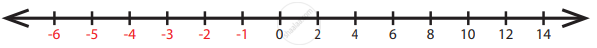Exercise 2.1 | Q 7. ii) | Page 33

From the following number line, identify the correct and the wrong representation with reason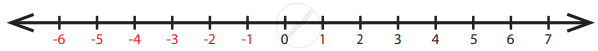Exercise 2.1 | Q 7. iii) | Page 33

From the following number line, identify the correct and the wrong representation with reason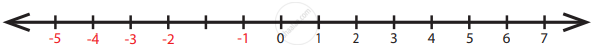Exercise 2.1 | Q 7. iv) | Page 33

From the following number line, identify the correct and the wrong representation with reason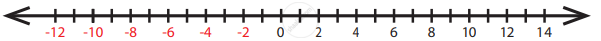Exercise 2.1 | Q 7. v) | Page 33

From the following number line, identify the correct and the wrong representation with reason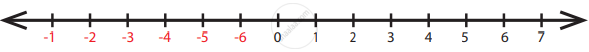Exercise 2.1 | Q 8. i) | Page 33

Write all the integers between the given numbers.

7 and 10

Exercise 2.1 | Q 8. ii) | Page 33

Write all the integers between the given numbers.

−5 and 4

Exercise 2.1 | Q 8. iii) | Page 33

Write all the integers between the given numbers.

−3 and 3

Exercise 2.1 | Q 8. iv) | Page 33

Write all the integers between the given numbers.

−5 and 0

Exercise 2.1 | Q 9. i) | Page 33

Put the appropriate signs as <, > or = in the box

−7 square 8

Exercise 2.1 | Q 9. ii) | Page 33

Put the appropriate signs as <, > or = in the box

−8 square −7

Exercise 2.1 | Q 9. iii) | Page 33

Put the appropriate signs as <, > or = in the box

−999 square −1000

Exercise 2.1 | Q 9. iv) | Page 33

Put the appropriate signs as <, > or = in the box

−111 square −111

Exercise 2.1 | Q 9. v) | Page 33

Put the appropriate signs as <, > or = in the box

0 square −200

Exercise 2.1 | Q 10. i) | Page 33

Arrange the following integers in ascending order

−11, 12, −13, 14, −15, 16, −17, 18, −19, −20

Exercise 2.1 | Q 10. ii) | Page 33

Arrange the following integers in ascending order.

−28, 6, −5, −40, 8, 0, 12, −1, 4, 22

Exercise 2.1 | Q 10. iii) | Page 33

Arrange the following integers in ascending order.

−100, 10, −1000, 100, 0, −1, 1000, 1, −10

Exercise 2.1 | Q 11. i) | Page 34

Arrange the following integers in descending order.

14, 27, 15, −14, −9, 0, 11, −17

Exercise 2.1 | Q 11. ii) | Page 34

Arrange the following integers in descending order.

−99, −120, 65, −46, 78, 400, −600

Exercise 2.1 | Q 11. iii) | Page 34

Arrange the following integers in descending order.

111, −222, 333, −444, 555, −666, 7777, −888

#### Objective Type Questions

Exercise 2.1 | Q 12 | Page 34

There are __________ positive integers from −5 to 6

• 5

• 6

• 7

• 11

Exercise 2.1 | Q 13 | Page 34

The opposite of 20 units to the left of 0 is

• 20

• 0

• −20

• 40

Exercise 2.1 | Q 14 | Page 34

One unit to the right of −7 is ___________

• +1

• −8

• −7

• −6

Exercise 2.1 | Q 15 | Page 34

3 units to the left of 1 is

• −4

• –3

• −2

• 3

Exercise 2.1 | Q 16 | Page 34

The number which determines marking the position of any number to its opposite on a number line is

• −1

• 0

• 1

• 10

Exercise 2.2 [Pages 34 - 36]

### Tamil Nadu Board Samacheer Kalvi solutions for Class 6th Mathematics Term 3 Answers Guide Chapter 2 IntegersExercise 2.2 [Pages 34 - 36]

#### Miscellaneous Practice Problems

Exercise 2.2 | Q 1 | Page 34

Write two different real life situations that represent the integer −3

Exercise 2.2 | Q 2. i) | Page 34

Mark the following numbers on a number line

All integers which are greater than −7 but less than 7

Exercise 2.2 | Q 2. ii) | Page 34

Mark the following numbers on a number line

The opposite of 3

Exercise 2.2 | Q 2. iii) | Page 34

Mark the following numbers on a number line

5 units to the left of −1

Exercise 2.2 | Q 3 | Page 34

Construct a number line that shows the depth of 10 feet from the ground level and its opposite

Exercise 2.2 | Q 4 | Page 34

Identify the integers and mark on the number line that are at a distance of 8 units from −6

Exercise 2.2 | Q 5 | Page 34

Answer the following questions from the number line given below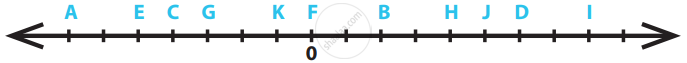i) Which integer is greater: G or K? Why?
ii) Find the integer that represents C.
iii) How many integers are there between G and H?
iv) Find the pairs of letters which are opposite of a number.
v) Say True or False: 6 units to the left of D is −6.

Exercise 2.2 | Q 6 | Page 35

If G is 3 and C is −1, what numbers are A and K on the number line?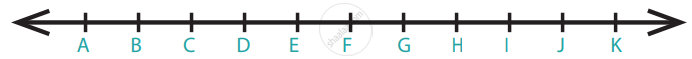Exercise 2.2 | Q 7 | Page 35

Find the integers that are 4 units to the left 0 and 2 units to the right of −3

#### Challenge Problems

Exercise 2.2 | Q 8 | Page 35

Is there the smallest and the largest number in the set of integers? Give reason

Exercise 2.2 | Q 9 | Page 35

Look at the Celsius Thermometer and answer the following questions:

i) What is the temperature that is shown in the Thermometer?
ii) Where will you mark the temperature 5°C below 0°C in the Thermometer?
iii) What will be the temperature if 10°C is reduced from the temperature shown in the Thermometer?
iv) Mark the opposite of 15°C in the Thermometer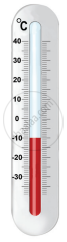Exercise 2.2 | Q 10 | Page 35

P, Q, R and S are four different integers on a number line. From the following clues, find these integers and write them in ascending order.

i) S is the least of the given integers.
ii) R is the smallest positive integer.
iii) The integers P and S are at the same distance from 0.
iv) Q is 2 units to the left of integer R.

Exercise 2.2 | Q 11 | Page 35

Assuming that the home to be the starting point, mark the following places in order on the number line as per instructions given below and write their corresponding integers.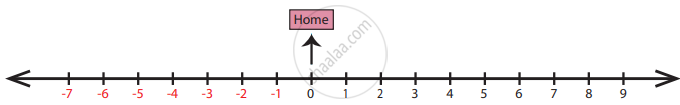Places: Home, School, Library, Playground, Park, Departmental Store, Bus Stand, Railway Station, Post Office, Electricity Board.
Instructions:
i) Bus Stand is 3 units to the right of Home.
ii) Library is 2 units to the left of Home.
iii) Departmental Store is 6 units to the left of Home.
iv) Post Office is 1 unit to the right of the Library.
v) Park is 1 unit right of Departmental Store.
vi) Railway Station is 3 units left of Post Office.
vii) Bus Stand is 8 units to the right of Railway Station.
viii) School is next to the right of Bus Stand.
ix) Playground and Library are opposite to each other.
x) Electricity Board and Departmental Store are at equal distance from Home.

Exercise 2.2 | Q 12 | Page 36

Complete the table using the following hints:
C1: the first non-negative integer.
C3: the opposite to the second negative integer.
C5: the additive identity in whole numbers.
C6: the successor of the integer in C2.
C8: the predecessor of the integer in C7.
C9: the opposite to the integer in C5.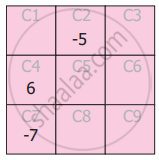Exercise 2.2 | Q 13 | Page 36

The following bar graph shows the profit (+) and loss (−) of a small scale company (in crores) between the years 2011 to 2017.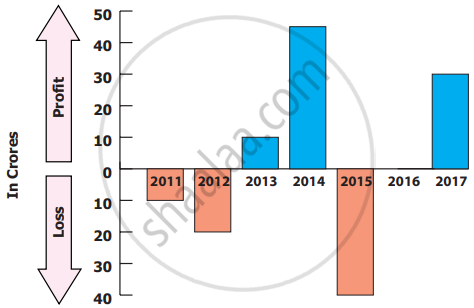i) Write the integer that represents a profit or a loss for the company in 2014?
ii) Denote by an integer on the profit or loss in 2016.
iii) Denote by integers on the loss for the company in 2011 and 2012.
iv) Say True or False: The loss is minimum in 2012.
v) Fill in: The amount of loss in 2011 is _______ as profit in 2013.

## Chapter 2: Integers

Exercise 2.1Exercise 2.2## Tamil Nadu Board Samacheer Kalvi solutions for Class 6th Mathematics Term 3 Answers Guide chapter 2 - Integers

Tamil Nadu Board Samacheer Kalvi solutions for Class 6th Mathematics Term 3 Answers Guide chapter 2 (Integers) include all questions with solution and detail explanation. This will clear students doubts about any question and improve application skills while preparing for board exams. The detailed, step-by-step solutions will help you understand the concepts better and clear your confusions, if any. Shaalaa.com has the Tamil Nadu Board of Secondary Education Class 6th Mathematics Term 3 Answers Guide solutions in a manner that help students grasp basic concepts better and faster.

Further, we at Shaalaa.com provide such solutions so that students can prepare for written exams. Tamil Nadu Board Samacheer Kalvi textbook solutions can be a core help for self-study and acts as a perfect self-help guidance for students.

Concepts covered in Class 6th Mathematics Term 3 Answers Guide chapter 2 Integers are Concept of Integers, Representation of Integers on the Number Line, Additive Inverse, Concept for Ordering of Integers.

Using Tamil Nadu Board Samacheer Kalvi Class 6th solutions Integers exercise by students are an easy way to prepare for the exams, as they involve solutions arranged chapter-wise also page wise. The questions involved in Tamil Nadu Board Samacheer Kalvi Solutions are important questions that can be asked in the final exam. Maximum students of Tamil Nadu Board of Secondary Education Class 6th prefer Tamil Nadu Board Samacheer Kalvi Textbook Solutions to score more in exam.

Get the free view of chapter 2 Integers Class 6th extra questions for Class 6th Mathematics Term 3 Answers Guide and can use Shaalaa.com to keep it handy for your exam preparation